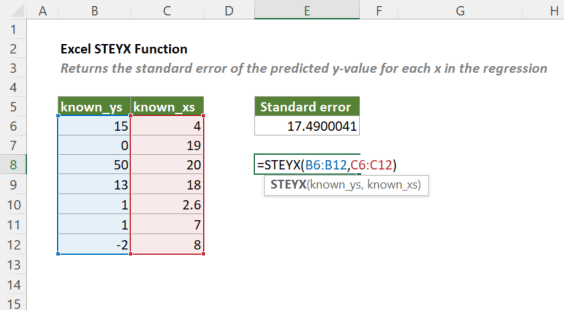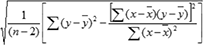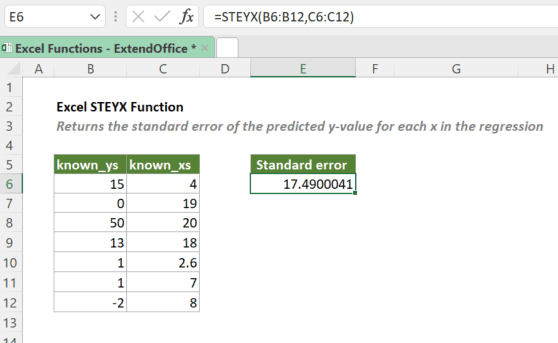## Excel STEYX Function

The STEYX function returns the standard error of the predicted y value for each x in a linear regression given by a series of coordinates represented by paired numbers.#### Syntax

=STEYX(known_ys, known_xs)

#### Arguments

• known_ys (required): An array or range of dependent variables.
• known_xs (required): An array or range of independent variables.

#### Return Value

The STEYX function returns a numeric value.

#### Function Notes

• Arguments can be either of the following:
• Numbers;
• Names, arrays, or references that contain numbers.
• Logical values and other values that can be converted to numbers that you type directly into the list of arguments will be counted.
• If text or empty cells are referenced as either of the arguments, the values will be ignored.
• STEYX returns the #N/A error if known_ys and known_xs have different numbers of data points.
• STEYX returns the #DIV/0! error if known_ys and known_xs are empty or have less than three data points.
• The equation for the standard error of the predicted y value is:Where x and y are the sample means of the two arrays of values, and n is the sample size.

#### Example

Take the table below as an example. To get the standard error of the predicted y-value for each x in the regression, please copy or enter the formula below in the result cell, and press Enter to get the result.

=STEYX(B6:B12,C6:C12)#### Related functions

Excel SLOPE Function

The SLOPE function returns the slope of a regression line based on the supplied y values and x values. The slope is the ratio of rise and run, which means the vertical distance divided by the horizontal distance between any two points on the line.

Excel INTERCEPT Function

The INTERCEPT function calculates the point at which a linear regression line will intersect the y-axis by using the given x-values and y-values.

Excel FORECAST Function

The FORECAST function predicts a future value based on existing values by using the linear regression.

### The Best Office Productivity Tools

#### Kutools for Excel - Helps You To Stand Out From Crowd

 Popular Features: Find, Highlight or Identify Duplicates  |  Delete Blank Rows  |  Combine Columns or Cells without Losing Data  |  Round without Formula ... Super VLookup: Multiple Criteria  |  Multiple Value  |  Across Multi-Sheets  |  Fuzzy Lookup... Adv. Drop-down List: Easy Drop Down List  |  Dependent Drop Down List  |  Multi-select Drop Down List... Column Manager: Add a Specific Number of Columns  |  Move Columns  |  Toggle Visibility Status of Hidden Columns  |  Compare Columns to Select Same & Different Cells ... Featured Features: Grid Focus  |  Design View  |  Big Formula Bar  |  Workbook & Sheet Manager | Resource Library (Auto Text)  |  Date Picker  |  Combine Worksheets  |  Encrypt/Decrypt Cells  |  Send Emails by List  |  Super Filter  |  Special Filter (filter bold/italic/strikethrough...) ... Top 15 Toolsets:  12 Text Tools (Add Text, Remove Characters ...)  |  50+ Chart Types (Gantt Chart ...)  |  40+ Practical Formulas (Calculate age based on birthday ...)  |  19 Insertion Tools (Insert QR Code, Insert Picture from Path ...)  |  12 Conversion Tools (Numbers to Words, Currency Conversion ...)  |  7 Merge & Split Tools (Advanced Combine Rows, Split Excel Cells ...)  |  ... and more

Kutools for Excel Boasts Over 300 Features, Ensuring That What You Need is Just A Click Away...#### Office Tab - Enable Tabbed Reading and Editing in Microsoft Office (include Excel)

• One second to switch between dozens of open documents!
• Reduce hundreds of mouse clicks for you every day, say goodbye to mouse hand.
• Increases your productivity by 50% when viewing and editing multiple documents.
• Brings Efficient Tabs to Office (include Excel), Just Like Chrome, Edge and Firefox.